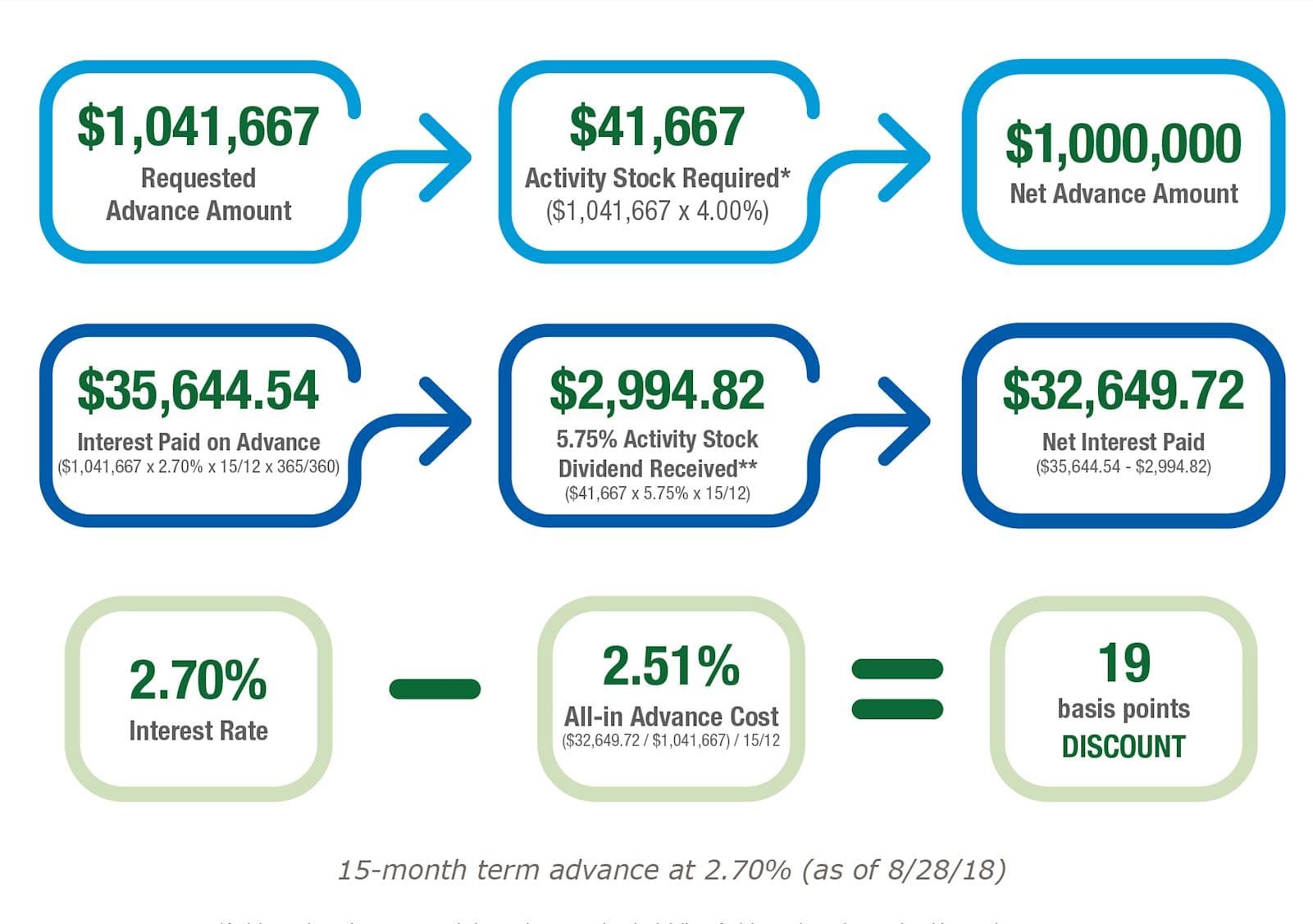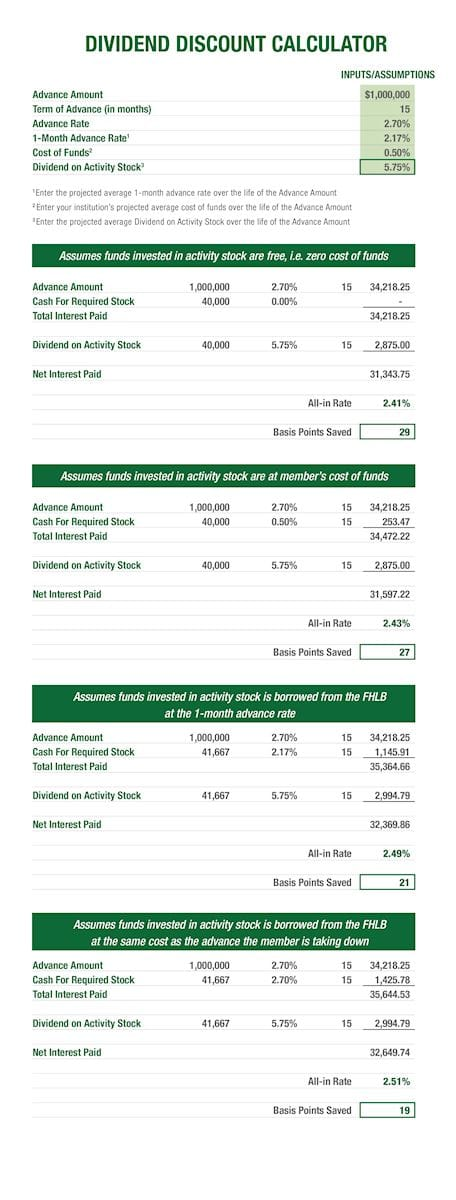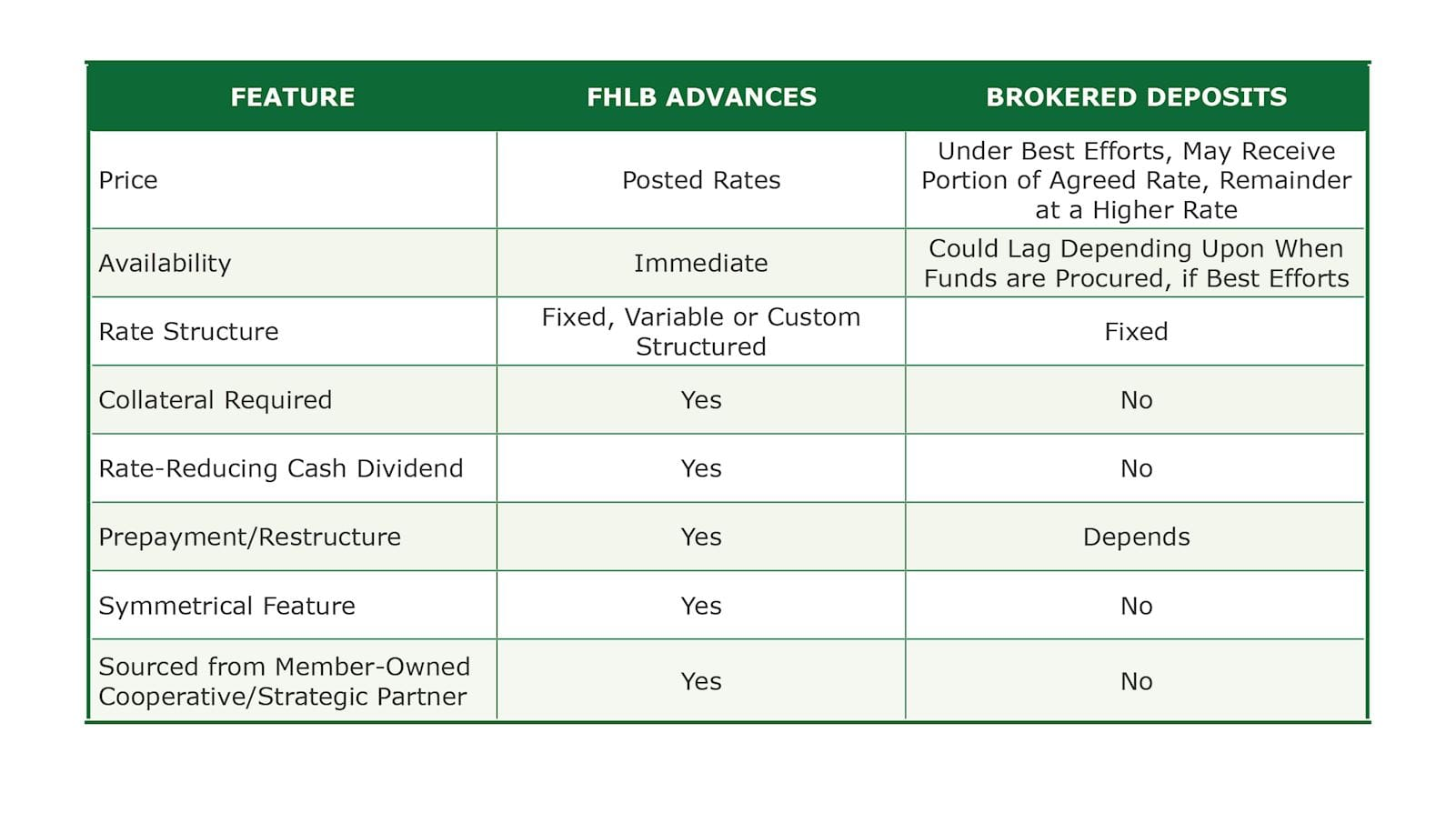# Raising Wholesale Funds: “Things are Seldom What They Seem”

posted on Monday, December 3, 2018 in Advances

## A Case of Comparing Funding Rates

Devotees of Gilbert and Sullivan may recall the line, “Things are seldom what they seem; skim milk masquerades as cream.” These cautionary words also apply to the need to dig below the surface when comparing rates on different sources of wholesale funding.

Consider the case of a community financial institution that wishes to raise \$1 million in the wholesale funding markets. There are two choices under consideration:

Posted Bullet 15-month FHLB Des Moines Bullet Advance: 2.70%
Posted Brokered 15-month CD: 2.60%

On the basis of rate, favoring the 15-month brokered CD would appear to be a no-brainer, right? Hold on.

## The Dividend Impact on All-In Wholesale Funding Rates

With the exception of Federal Home Loan Bank advances, other sources of wholesale funding do not entail investments or receipt of cash dividends. For example, the Federal Home Loan Bank of Des Moines currently requires its members to invest 4.00% of drawn advances in the form of activity-based stock. This class of stock currently supports an annualized dividend rate of 5.75%. A financial institution could assign a cost of funding the activity-based stock that would likely be below the dividend return. The cost of funding the activity-based stock could, as an example, be represented by such assumed benchmarks as a hurdle rate of capital or an alternative investment. Effectively, this spread could be thought of as cash consideration that could be applied as a discount to a posted advance rate.

Going back to the example of a 15-month posted advance rate of 2.70%, let’s build a case for estimating the impact of the positive spread generated on the activity stock investment, per Figure 1.Figure 1: Derivation of Discount on Advance Rate Derived from Dividend Yield Spread

With collective assumptions (we’ll get to them in a minute), we can see that in order to derive \$1,000,000 in net advance proceeds, 4.00% of the grossed-up \$1,041,667, or \$41,667, would be required as a purchase of activity stock. Once cash dividends on the activity stock are deducted from interest paid on the advance, \$32,649.72 would represent the net payment amount. Dividing by the gross amount of advances, we can assume that the all-in advance rate is 2.51%, rather than the posted level of 2.70%. The yield is nine basis points below that of the brokered CD with a comparable maturity.

Now for the assumptions embedded within the above calculation. First, the methodology assumes that the level of the 5.75% dividend will remain static for the life of the 15-month advance. The dividend rate can vary. Hence, we might consider projecting the average dividend on activity stock over the life of the advance. Second, we have assumed that a borrower’s cost of funds is invested in the required activity stock. So, depending on the borrower’s preference, there are effectively four differing assumptions that can be made in terms of the cost of investing funds in FHLB Des Moines activity stock: invested funds:

Zero cost. This option may be less of a reasonable assumption, especially in the current rising rate environment.

Funds invested in FHLB Des Moines activity stock are equivalent to the borrower’s general cost of funds.

Assignment of a specific funding cost benchmark such as the federal funds, a specific advance rate or a borrower’s cost of deposits. Ideally, if a borrower were to choose a specific cost benchmark, the duration of the benchmark would ideally coincide with that of the activity stock.

Invested funds are sourced from FHLB Des Moines at the same cost as the advance. Such an assumption would suppose that a borrower uses a portion of the financing to purchase the required activity stock.

With the assistance of a dividend calculator available from FHLB Des Moines, Figure 2. demonstrates that, depending upon which cost of funds assumption is used, assuming a static dividend rate of 5.75%, the discount from posted advance levels would range between 19 basis points (as is assumed in Figure 1.) to 29 basis points.Figure 2. Discount Off of Posted Advance Rates Using Various Cost of Funds Assumptions

## A Dividend-Adjusted Comparison of Wholesale Funding Sources

Under the more conservative cost of funding assumption (where funds invested in activity stock are assumed to be sourced at the same rate as the advance), the all-in rate on the 15-month bullet advance is calculated at 2.51%, versus the posted brokered CD rate of 2.60%. Aside from the adjusted rate comparison, there are qualitative differences between the two funding sources that may be highlighted, as illustrated in Figure 3.Figure 3.  Key Feature Differences Advances vs. Brokered CD’s

When it comes to wholesale funding rates, what you see may not necessarily be what you get! Depending upon the interest rate environment or location on the yield curve, dividend-adjusted advance rates may or may not be comparable. You’ll never know unless you run the dividend adjustment calculation.

Run your own customized scenario by accessing the tool below:

TAGS